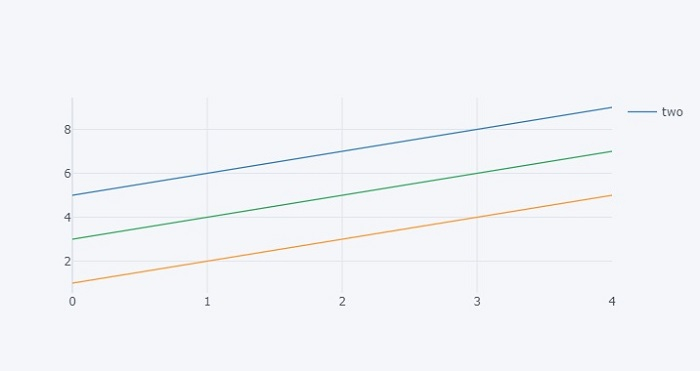# Python Plotly ŌĆō How to hide legend entries in a Plotly figure?

Plotly is an open-source plotting library in Python that can generate several different types of charts. Python users can use Plotly to create interactive web-based visualizations including scientific charts, 3D graphs, statistical charts, financial charts, etc. Plotly can also be used in static document publishing and desktop editors such as PyCharm and Spyder.

In this tutorial, we will show how you can use Plotly to hide legend entries for a particular field. Follow the steps given below to hide the legend entries.

Step 1

Import the plotly.offline module and alias as py.

import plotly.offline as py


Step 2

Import the plotly.graphs_objs module and alias as go.

import plotly.graphs_objs as go


Step 3

Create a dataset with the following values ŌłÆ

data = {
'one':[1,2,3,4,5],
'two':[5,6,7,8,9],
'three':[3,4,5,6,7]
}
df = pd.DataFrame(data)


Step 4

Create a scatter plot as follows ŌłÆ

# generate scatter plot
fig = df.iplot(kind='scatter', asFigure=True)


Step 5

Set the "for" loop and "if" condition to hide the legend for the first and third columns.

# set for loop for figure data
for trace in fig['data']:
# Hide legend column names not equal to 'two'
if(trace['name'] != 'two'): trace['showlegend'] = False


## Example

The complete code to hide legend entries is as follows ŌłÆ

import pandas as pd
import plotly.offline as py
cf.go_offline()

# Create a dataset
data = {
'one':[1,2,3,4,5],
'two':[5,6,7,8,9],
'three':[3,4,5,6,7]
}

# Generate dataframe
df = pd.DataFrame(data)

# generate scatter plot
fig = df.iplot(kind='scatter', asFigure=True)

# set for loop for figure data
for trace in fig['data']:

# hide legend column names not equal to 'two'
if(trace['name'] != 'two'): trace['showlegend'] = False

# generate webpage
py.plot(fig)


## Output

It will show the following output on the browser ŌłÆObserve that the legend entries for the first two lines are hidden.

Updated on: 21-Oct-2022

2K+ Views